Download Presentation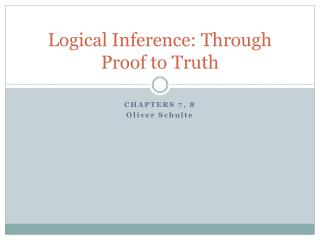Logical Inference: Through Proof to Truth

# Logical Inference: Through Proof to Truth - PowerPoint PPT Presentation

Logical Inference: Through Proof to Truth. CHAPTERS 7, 8 Oliver Schulte. Active Field: Automated Deductive Proof. Call for Papers. Proof methods. Proof methods divide into (roughly) two kinds: Application of inference rules: Legitimate (sound) generation of new sentences from old.I am the owner, or an agent authorized to act on behalf of the owner, of the copyrighted work described.
Download Presentation## Logical Inference: Through Proof to Truth

An Image/Link below is provided (as is) to download presentation

Download Policy: Content on the Website is provided to you AS IS for your information and personal use and may not be sold / licensed / shared on other websites without getting consent from its author.While downloading, if for some reason you are not able to download a presentation, the publisher may have deleted the file from their server.

- - - - - - - - - - - - - - - - - - - - - - - - - - E N D - - - - - - - - - - - - - - - - - - - - - - - - - -
Presentation Transcript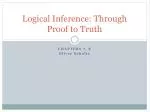### Logical Inference: Through Proof to Truth

CHAPTERS 7, 8

Oliver Schulte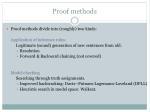Proof methods
• Proof methods divide into (roughly) two kinds:

Application of inference rules:

Legitimate (sound) generation of new sentences from old.

• Resolution
• Forward & Backward chaining (not covered)

Model checking

Searching through truth assignments.

• Improved backtracking: Davis--Putnam-Logemann-Loveland (DPLL)
• Heuristic search in model space: Walksat.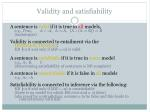Validity and satisfiability

A sentence is valid if it is true in all models,

e.g., True, A A, A  A, (A  (A  B))  B

(tautologies)

Validity is connected to entailment via the Deduction Theorem:

KB ╞ α if and only if (KB α) is valid

A sentence is satisfiable if it is true in some model

e.g., A B, C

(determining satisfiability of sentences is NP-complete)

A sentence is unsatisfiable if it is false in all models

e.g., AA

Satisfiability is connected to inference via the following:

KB ╞ α if and only if (KBα) is unsatisfiable

(there is no model for which KB=true and a is false)

(aka proof by contradiction: assume a to be false and this leads to contradictions with KB)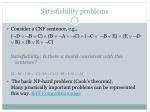Satisfiability problems
• Consider a CNF sentence, e.g.,

(D B  C)  (B A C)  (C B  E)  (E D  B)  (B  E C)

Satisfiability: Is there a model consistent with this sentence?

[A  B]  [¬B  ¬C]  [A  C]  [¬D]  [¬D  ¬A]

• The basic NP-hard problem (Cook’s theorem). Many practically important problems can be represented this way. SAT Competition page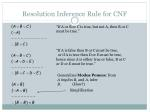Resolution Inference Rule for CNF

“If A or B or C is true, but not A, then B or C must be true.”

“If A is false then B or C must be true,

or if A is true then D or E must be true, hence since A is either true or false, B or C or D or E must be true.”

Generalizes Modus Ponens: fromA implies B, and A, inferB. (How?)

Simplification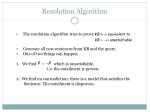Resolution Algorithm
• The resolution algorithm tries to prove:
• Generate all new sentences from KB and the query.
• One of two things can happen:
• We find which is unsatisfiable,
• i.e. the entailment is proven.
• 2. We find no contradiction: there is a model that satisfies the
• Sentence. The entailment is disproven.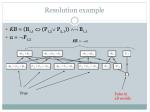Resolution example
• KB = (B1,1 (P1,2 P2,1))  B1,1
• α = P1,2

True

False in

all worlds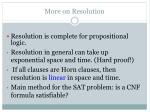More on Resolution
• Resolution is complete for propositional logic.
• Resolution in general can take up exponential space and time. (Hard proof!)
• If all clauses are Horn clauses, then resolution is linear in space and time.
• Main method for the SAT problem: is a CNF formula satisfiable?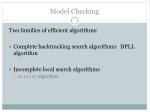Model Checking

Two families of efficient algorithms:

• Complete backtracking search algorithms: DPLL algorithm
• Incomplete local search algorithms
• WalkSAT algorithmThe DPLL algorithm

Determine if an input propositional logic sentence (in CNF) is

satisfiable. This is just like backtracking search for a CSP.

Improvements:

• Early termination

A clause is true if any literal is true.

A sentence is false if any clause is false.

• Pure symbol heuristic

Pure symbol: always appears with the same "sign" in all clauses.

e.g., In the three clauses (A B), (B C), (C  A), A and B are pure, C is impure.

Make a pure symbol literal true.

(if there is a model for S, then making a pure symbol true is also a model).

3 Unit clause heuristic

Unit clause: only one literal in the clause

The only literal in a unit clause must be true.

In practice, takes 80% of proof time.

Note: literals can become a pure symbol or a

unit clause when other literals obtain truth values. e.g.The WalkSAT algorithm
• Incomplete, local search algorithm
• Begin with a random assignment of values to symbols
• Each iteration: pick an unsatisfied clause
• Flip the symbol that maximizes number of satisfied clauses, OR
• Flip a symbol in the clause randomly
• Trades-off greediness and randomness
• Many variations of this idea
• If it returns failure (after some number of tries) we cannot tell whether the sentence is unsatisfiable or whether we have not searched long enough
• If max-flips = infinity, and sentence is unsatisfiable, algorithm never terminates!
• Typically most useful when we expect a solution to existHard satisfiability problems
• Consider random 3-CNF sentences. e.g.,

(D B  C)  (B A C)  (C B  E)  (E D  B)  (B  E C)

m = number of clauses (5)

n = number of symbols (5)

• Underconstrained problems:
• Relatively few clauses constraining the variables
• Tend to be easy
• 16 of 32 possible assignments above are solutions
• (so 2 random guesses will work on average)Hard satisfiability problems
• What makes a problem hard?
• Increase the number of clauses while keeping the number of symbols fixed
• Problem is more constrained, fewer solutions
• Investigate experimentally….Run-time for DPLL and WalkSAT
• Median runtime for 100 satisfiable random 3-CNF sentences, n = 50Summary
• Determining the satisfiability of a CNF formula is the basic problem of propositional logic (and of many reasoning/scheduling problems).
• Resolution is complete for propositional logic.
• Can use search methods + inference (e.g. unit propagation): DPLL.
• Can also use stochastic local search methods: WALKSAT.